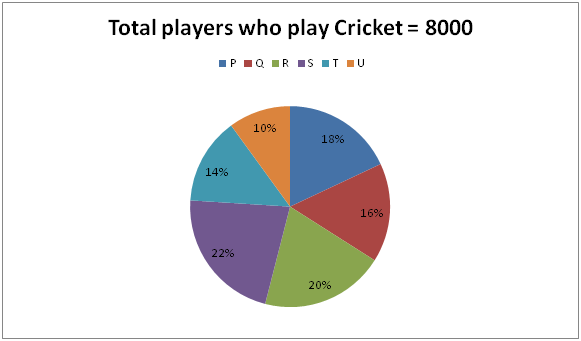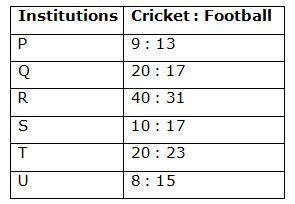# LIC HFL Associate/ Assistant Numerical Ability Questions – (Day-02)

Dear Aspirants, Our IBPS Guide team is providing new series of Numerical Ability Questions for LIC HFL Associate/ Assistant 2019 so the aspirants can practice it on a daily basis. These questions are framed by our skilled experts after understanding your needs thoroughly. Aspirants can practice these new series questions daily to familiarize with the exact exam pattern and make your preparation effective.

Check here for LIC HFL Mock Test 2019

Check here for IBPS RRB PO Mains Mock Test 2019

Check here for IBPS RRB Clerk Mains Mock Test 2019

### Click Here to Subscribe Crack High Level Puzzles & Seating Arrangement Questions PDF 2019 Plan

[WpProQuiz 6914]

Directions (1 – 5): Study the following information carefully and answer the given questions.

The following pie chart shows the percentage distribution of total number of players who play cricket in 6 different institutions.The following table shows the ratio of total number of players who play cricket and football in those institutions.1) Find the ratio between the total number of players who play football in institute P to that of institute S.

a) 156: 193

b) 123: 172

c) 134: 147

d) 75: 68

e) None of these

2) Find the difference between the total number of players who play Cricket in institute Q and R together to that of total number of players who play football in institute Q and T together.

a) 504

b) 556

c) 623

d) 678

e) None of these

3) Total number of players who play Cricket in institute P and S together is approximately what percentage of total number of players who play Football in institute R and U together?

a) 146 %

b) 155 %

c) 103 %

d) 117 %

e) 135 %

4) Find the average number of players who play football in all the given institutions except institute T.

a) 1840

b) 1780

c) 1960

d) 2050

e) None of these

5) Find the difference between the average number of players who play cricket in institute T and U together to that of the average number of players who play football in institute Q and T together.

a) 352

b) 275

c) 376

d) 413

e) None of these

6) A vessel of 120 liters contains milk and water. If 60 % of milk and 30 % of water is taken out, then the vessel will be half empty. Find the ratio of initial quantity of milk and water in the vessel?

a) 3 : 2

b) 5 : 3

c) 7 : 5

d) 2 : 1

e) None of these

7) How many 6 letter words with or without meaning can be formed out of the letters of the word, ‘PRECAUTION’, if repetition of letters is not allowed?

a) 380

b) 2840

c) 151200

d) 56600

e) None of these

8) A man drives 360 km from A to B in 5 hours and returns to B in 4 hours. If M is the average speed of the entire trip, then the average speed for the journey from B to A exceeds M by?

a) 10 km/hr

b) 16 km/hr

c) 12 km/hr

d) 8 km/hr

e) None of these

9) A box contains the coins of 10p, 50p and 1 rupee is in the ratio of      5: 4: 3. If there is Rs. 22 in all, then find the total number of 50p coins?

a) 14

b) 16

c) 12

d) 18

e) None of these

10) A train crosses a pole in 25 sec and the same train crosses a platform of length 150 m in 40 sec. Find the length of the train?

a) 200 m

b) 250 m

c) 150 m

d) 400 m

e) None of these

Direction (1-5) :

The total number of players who play football in institute P

= >8000 * (18/100) * (13/9) = 2080

The total number of players who play football in institute S

= >8000 * (22/100) * (17/10) = 2992

Required ratio = 2080 : 2992 = 130 : 187

The total number of players who play Cricket in institute Q and R together

= >8000 * (36/100) = 2880

The total number of players who play football in institute Q and T together

= >8000 * (16/100) * (17/20) + 8000 * (14/100) * (23/20)

= >1088 + 1288 = 2376

Required difference = 2880 – 2376 = 504

Total number of players who play Cricket in institute P and S together

= > 8000 * (40/100) = 3200

Total number of players who play Football in institute R and U together

= >8000 * (20/100) * (31/40) + 8000 * (10/100) * (15/8)

= >1240 + 1500 = 2740

Required % = (3200 / 2740) * 100 = 116.788 % = 117 %

The total number of players who play football in all the given institutions except institute T

= > (8000 * (18/100) * (13/9) + 8000 * (16/100) * (17/20) + 8000 * (20/100) * (31/40) + 8000 * (22/100) * (17/10) + 8000 * (10/100) * (15/8))

= > (2080 + 1088 + 1240 + 2992 + 1500) = 8900

Required average = 8900 / 5 = 1780

The average number of players who play cricket in institute T and U together

= >8000 * (24/100) * (1/2) = 960

The average number of players who play football in institute Q and T together

= >[8000 * (16/100) * (17/20) + 8000 * (14/100) * (23/20)] / 2

= > (1088 + 1288) / 2 = 1188

Required difference = 1188 – 960 = 228

Direction (6-10) :

M + W = 120 -à (1)

(60/100) * M + (30/100) * W = 60

6M + 3W = 600 —> (2)

By solving the equation (1) and (2), we get,

M = 80 liters, W = 40 liters

Required ratio = 80 : 40 = 2 : 1

‘PRECAUTION’ contains 10 different letters.

Required number of words = 10p6

= > (10 * 9 * 8 * 7 * 6 * 5) = 151200

The speed from A to B = 360/5 = 72 km/hr

The speed from B to A = 360/4 = 90 km/hr

The average speed of the entire trip = 2xy/(x+ y)

= > [(2 * 72 * 90) / 162]

= > 80 km/hr

Required difference = 90 – 80 = 10 km/hr

A box contains the coins of 10p, 50p and 1 rupee is in the ratio = 5: 4: 3 (5x, 4x, 3x)

22 = [(5x * 10) / 100] + [(4x * 50) / 100] + [(3x * 1)]

22 = (50x/100) + (200x/100) + 3x

22 = (x/2) + 2x + 3x

44 = 11x

x = 4

The total number of 50p coins = 4x = 16

Let the length of train be x,

Here, the speed of the train is equal. So,

(x / 25) = (x + 150) / 40

8x = 5x + 750

3x = 750

x = 250

The length of the train (x) = 250 m